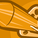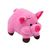App Development

Announcements
QlikWorld 2022, LIVE in Denver CO., May 16-19, 2022. REGISTER NOW TO RECEIVE EARLY BIRD PRICING
cancel
Showing results for
Did you mean:Contributor II

Convert Measure to Dimension

I have this if statement that I wish to use as a dimension.

=if(Count(distinct AssessmentId) <= 4, 'Low Risk',
if(Count(distinct AssessmentId) > 4 and Count(distinct AssessmentId) <= 10, 'Medium Risk',
'High Risk'))

Currently, it can only be used as a measure kindly assist me in converting it to a Dimension

Labels (3)

• IF AND STATEMENT

7 RepliesPartner

You need to provide at least one other dimension via aggr()-formula to show where the distinct Id's should be counted.
In the example below I added a fictional  "CustomerID" field for demonstration. Simply replace this field with one or more dimensions.

=
if(AGGR(COUNT(DISTINCT AssessmentId),CustomerID) <= 4, 'Low Risk',
if(AGGR(COUNT(DISTINCT AssessmentId),CustomerID) > 4 and AGGR(COUNT(DISTINCT AssessmentId),CustomerID) <= 10, 'Medium Risk',
'High Risk'))PartnerContributor II
Author

This just returns High riskPartner

Hi,

Can you share a sample dataMVP

Whilst you can use calculations as dimensions it is not generally a good idea, and the selections can be a bit weird.

The best bet is to pre-aggregate the data on the load, so that you have a field which can then be used as a dimension.

What you don't mention above is what the dimension is that you are aggregating over. I shall assume it's PolicyID for this example.

Insert this code after the load of your main table, to do the aggregation:

RiskStatus:
PolicyID,
if(Count(distinct AssessmentId) <= 4, 'Low Risk',
if(Count(Count(distinct AssessmentId) <= 10, 'Medium Risk',
'High Risk')) as [Risk Rating],
count(distinct AssessmentId) as Assessments
RESIDENT ExistingTable
GROUP BY PolicyID;

The thing to bear in mind (and this may dictate whether this approach works for you) is that the Risk Rating will not change based on selections. With the code in the front end, selecting an AssessmentId will make the result automatically Low Risk, by calculating it in the load script selections will not have an impact.

Hope that helps.Specialist

Hi,

just you can add under the field of "distinct AssessmentId" in Main table,

if(Count(distinct AssessmentId) <= 4, 'Low Risk',
if(Count(distinct AssessmentId) > 4 and Count(distinct AssessmentId) <= 10, 'Medium Risk',
'High Risk')) as Risk_Status,

then you can call Risk_Status field as Dimension in chart or table.

=if(Count(distinct AssessmentId) <= 4, 'Low Risk',
if(Count(distinct AssessmentId) > 4 and Count(distinct AssessmentId) <= 10, 'Medium Risk',
'High Risk'))Contributor III